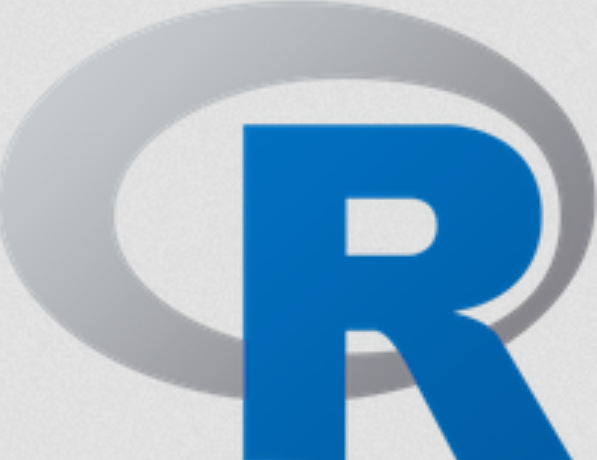# Linear Regression from scratch in ROne of the very first learning algorithms that you’ll encounter when studying data science and machine learning is least squares linear regression. Linear regression is one of the easiest learning algorithms to understand; it’s suitable for a wide array of problems, and is already implemented in many programming languages.

Most users are familiar with the lm() function in R, which allows us to perform linear regression quickly and easily. But one drawback to the lm() function is that it takes care of the computations to obtain parameter estimates (and many diagnostic statistics, as well) on its own, leaving the user out of the equation. So, how can we obtain these parameter estimates from scratch?

In this post, I will outline the process from first principles in R. I will use only matrices, vectors, and matrix operations to obtain parameter estimates using the closed-form linear algebraic solution. After reading this post, you’ll see that it’s actually quite simple, and you’ll be able to replicate the process with your own data sets (though using the lm() function is of course much more efficient and robust).## Share:

Subscribe
Notify ofInline Feedbacks

On social media